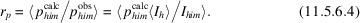International
Tables for
Crystallography
Volume F
Crystallography of biological macromolecules
Edited by E. Arnold, D. M. Himmel and M. G. Rossmann

International Tables for Crystallography (2012). Vol. F, ch. 11.5, p. 298   | 1 | 2 |

## Section 11.5.6. Estimating the quality of data scaling and averaging

C. G. van Beek,a R. Bolotovskya§ and M. G. Rossmanna*

aDepartment of Biological Sciences, Purdue University, West Lafayette, IN 47907–1392, USA
Correspondence e-mail:  mgr@indiana.bio.purdue.edu

### 11.5.6. Estimating the quality of data scaling and averaging

| top | pdf |

A commonly used estimate of the quality of scaled and averaged Bragg reflection intensities is. Useful definitions of R factors are:The linear (R1), square (R2) and weighted () R factors can be subdivided into resolution ranges, intensity ranges, reflection classes, frame number and regions of the detector surface. When method 1is used, reflectionscan be grouped in terms of the sums of partialities of contributing partial reflections.

The R-factor variation depends on the properties of the detector with respect to intensities. Generally the R factor decreases as intensity increases. Thus, the R factor generally increases with resolution. Any deviation from this behaviour might indicate a problem in the data collection due to non­linearity of the detector response, ice diffuse diffraction, or any other stray effects superimposed on the crystal diffraction.

A useful indicator of the quality of the intensity estimates of partial reflections is the mean ratio of calculated partiality to observed partiality:The deviation of this ratio from unity can be examined as a function of the reflection intensity, resolution and calculated partiality.

The comparison of R factors for centric and noncentric reflections can be used to determine the significance of an anomalous-scattering effect. The quality of the anomalous-dispersion signal can be assessed by calculation of the scatter,, whereandis the average of the n measurements of the full reflection intensities. Thevalues for noncentric reflections can be compared to the scatter,or, of reflections differing only in absorption while excluding Bijvoet opposites. The mean scatter is calculated from allvalues,The ratiosandshould be larger than unity for significant anomalous-dispersion data.